Скачать презентацию Intermolecular Forces Liquids and Solids Chapter 11

3514fd51028d3e1161ba419cfc4288a4.ppt

• Количество слайдов: 38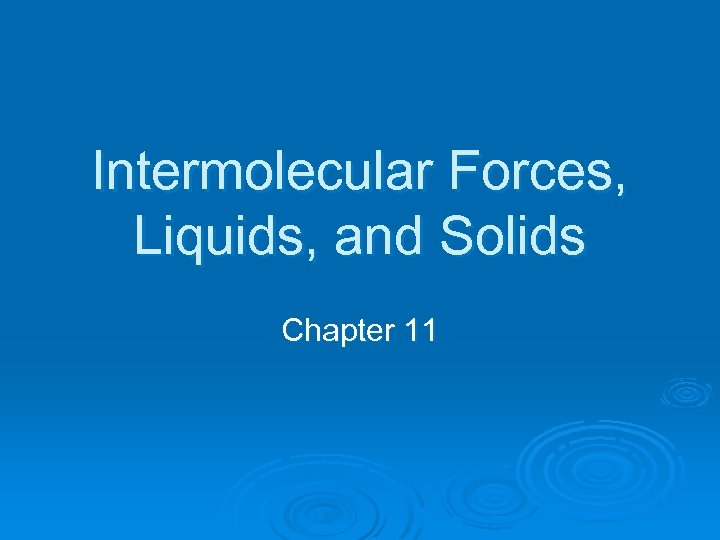Intermolecular Forces, Liquids, and Solids Chapter 11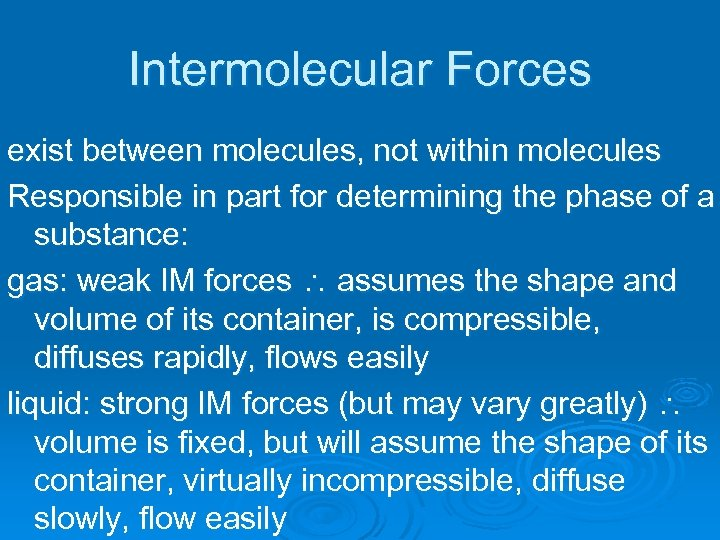Intermolecular Forces exist between molecules, not within molecules Responsible in part for determining the phase of a substance: gas: weak IM forces assumes the shape and volume of its container, is compressible, diffuses rapidly, flows easily liquid: strong IM forces (but may vary greatly) volume is fixed, but will assume the shape of its container, virtually incompressible, diffuse slowly, flow easily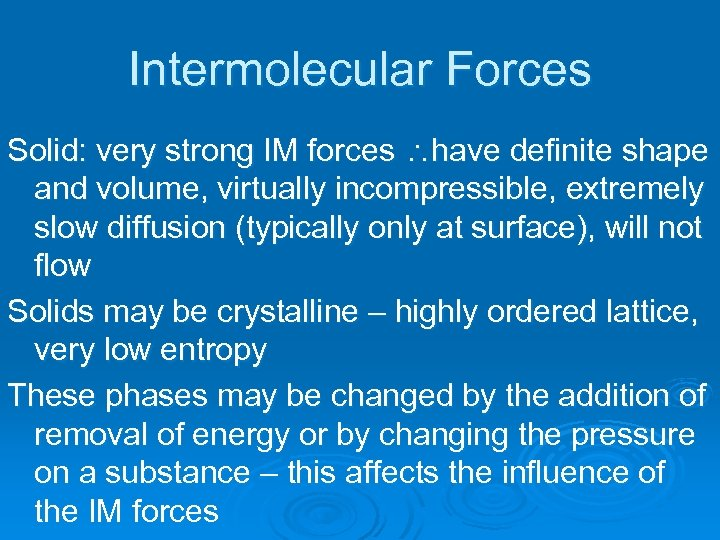Intermolecular Forces Solid: very strong IM forces have definite shape and volume, virtually incompressible, extremely slow diffusion (typically only at surface), will not flow Solids may be crystalline – highly ordered lattice, very low entropy These phases may be changed by the addition of removal of energy or by changing the pressure on a substance – this affects the influence of the IM forces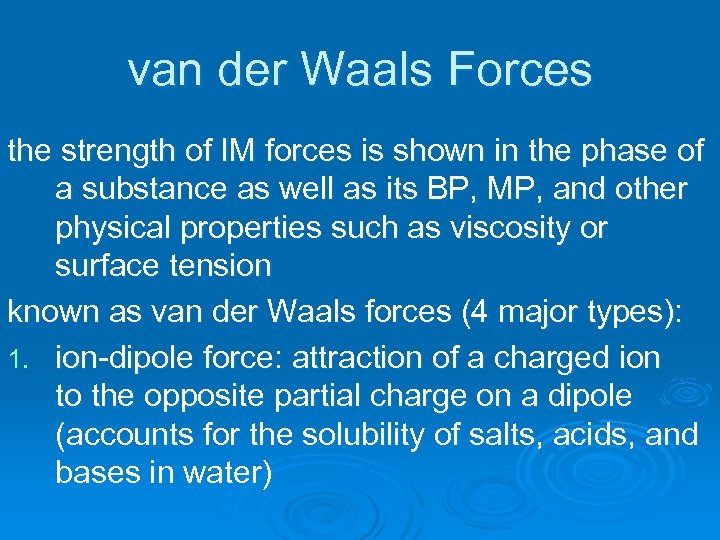van der Waals Forces the strength of IM forces is shown in the phase of a substance as well as its BP, MP, and other physical properties such as viscosity or surface tension known as van der Waals forces (4 major types): 1. ion-dipole force: attraction of a charged ion to the opposite partial charge on a dipole (accounts for the solubility of salts, acids, and bases in water)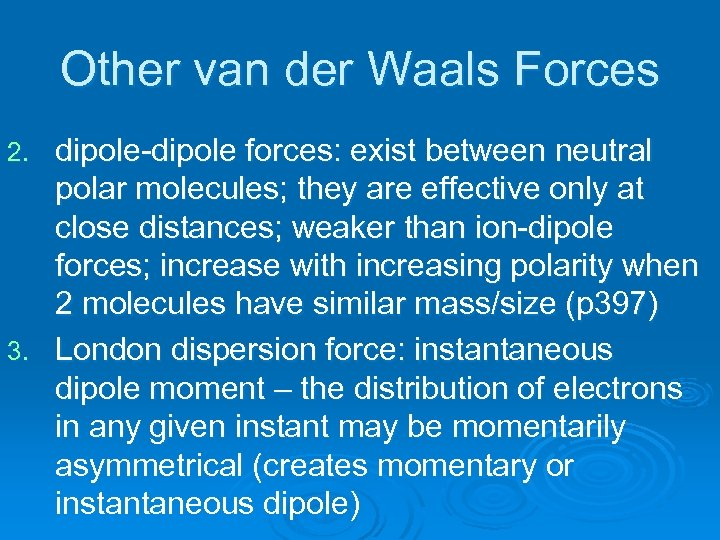Other van der Waals Forces dipole-dipole forces: exist between neutral polar molecules; they are effective only at close distances; weaker than ion-dipole forces; increase with increasing polarity when 2 molecules have similar mass/size (p 397) 3. London dispersion force: instantaneous dipole moment – the distribution of electrons in any given instant may be momentarily asymmetrical (creates momentary or instantaneous dipole) 2.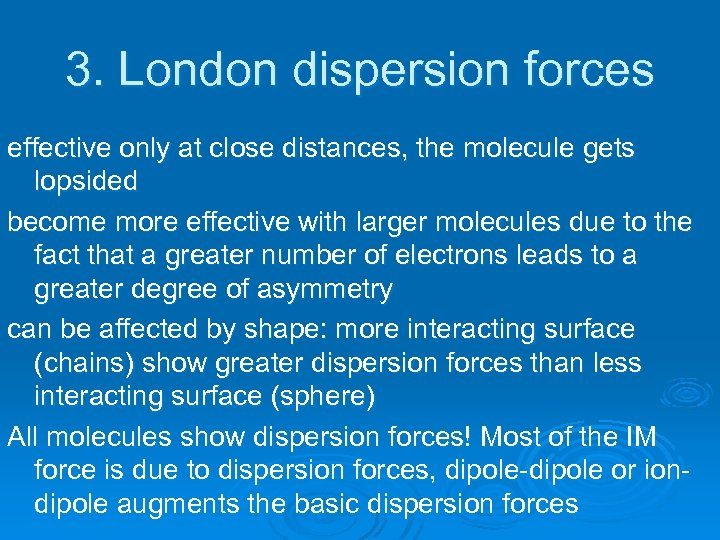3. London dispersion forces effective only at close distances, the molecule gets lopsided become more effective with larger molecules due to the fact that a greater number of electrons leads to a greater degree of asymmetry can be affected by shape: more interacting surface (chains) show greater dispersion forces than less interacting surface (sphere) All molecules show dispersion forces! Most of the IM force is due to dispersion forces, dipole-dipole or iondipole augments the basic dispersion forces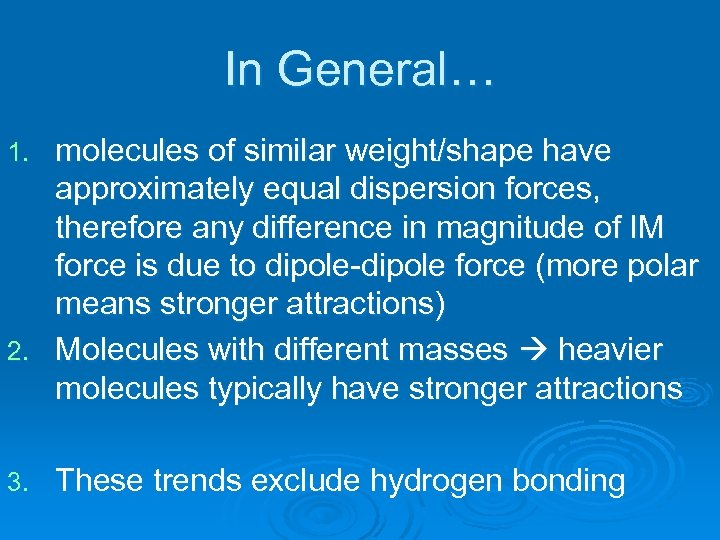In General… molecules of similar weight/shape have approximately equal dispersion forces, therefore any difference in magnitude of IM force is due to dipole-dipole force (more polar means stronger attractions) 2. Molecules with different masses heavier molecules typically have stronger attractions 1. 3. These trends exclude hydrogen bonding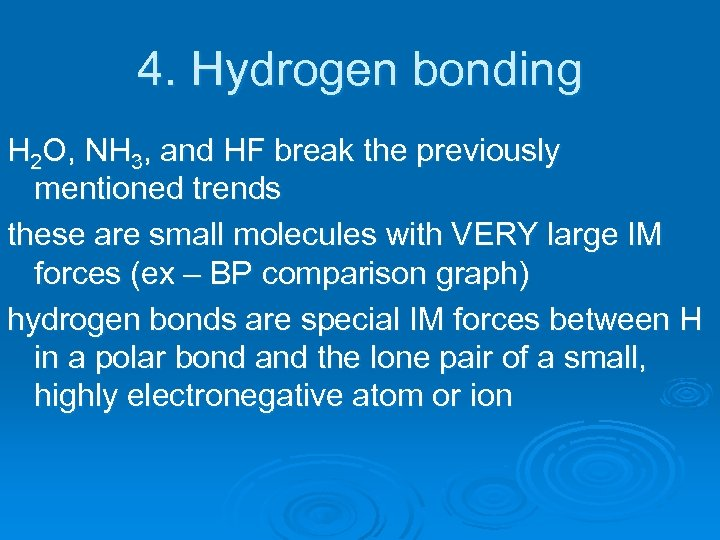4. Hydrogen bonding H 2 O, NH 3, and HF break the previously mentioned trends these are small molecules with VERY large IM forces (ex – BP comparison graph) hydrogen bonds are special IM forces between H in a polar bond and the lone pair of a small, highly electronegative atom or ion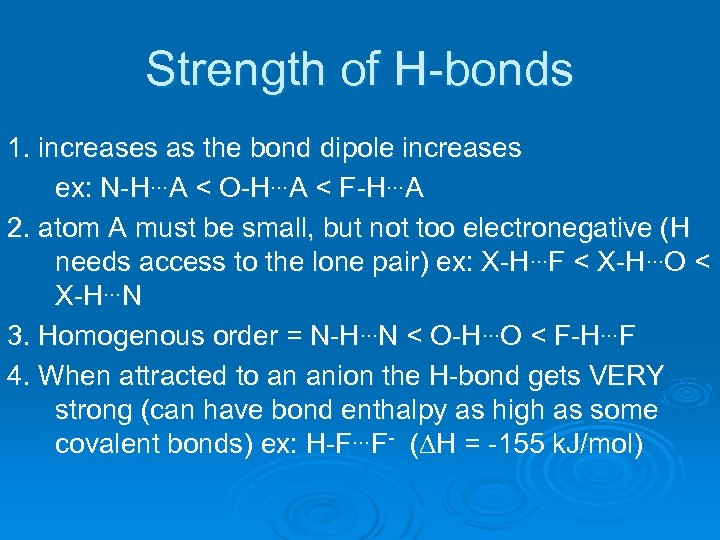Strength of H-bonds 1. increases as the bond dipole increases ex: N-H…A < O-H…A < F-H…A 2. atom A must be small, but not too electronegative (H needs access to the lone pair) ex: X-H…F < X-H…O < X-H…N 3. Homogenous order = N-H…N < O-H…O < F-H…F 4. When attracted to an anion the H-bond gets VERY strong (can have bond enthalpy as high as some covalent bonds) ex: H-F…F- (DH = -155 k. J/mol)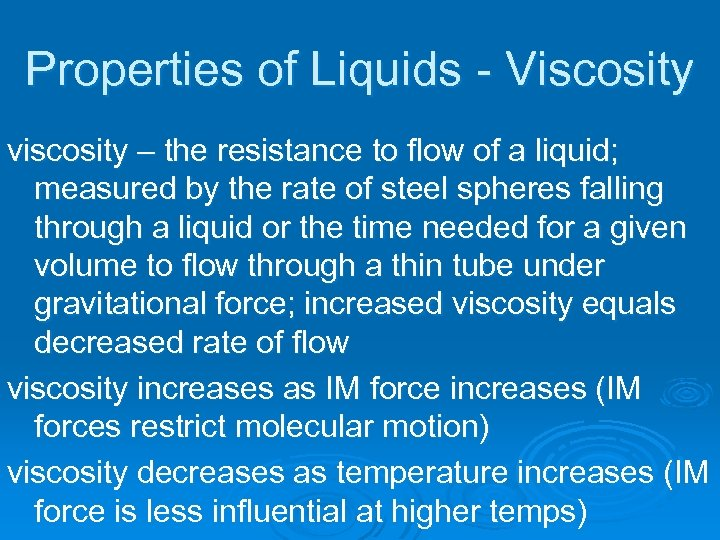Properties of Liquids - Viscosity viscosity – the resistance to flow of a liquid; measured by the rate of steel spheres falling through a liquid or the time needed for a given volume to flow through a thin tube under gravitational force; increased viscosity equals decreased rate of flow viscosity increases as IM force increases (IM forces restrict molecular motion) viscosity decreases as temperature increases (IM force is less influential at higher temps)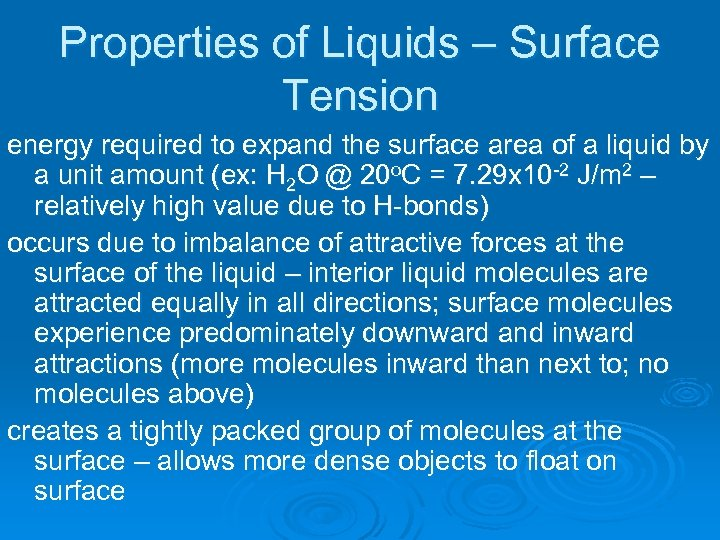Properties of Liquids – Surface Tension energy required to expand the surface area of a liquid by a unit amount (ex: H 2 O @ 20 o. C = 7. 29 x 10 -2 J/m 2 – relatively high value due to H-bonds) occurs due to imbalance of attractive forces at the surface of the liquid – interior liquid molecules are attracted equally in all directions; surface molecules experience predominately downward and inward attractions (more molecules inward than next to; no molecules above) creates a tightly packed group of molecules at the surface – allows more dense objects to float on surface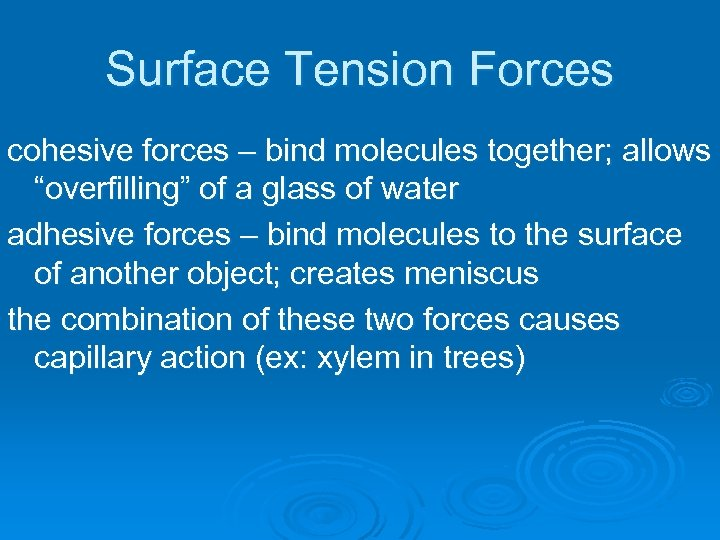Surface Tension Forces cohesive forces – bind molecules together; allows “overfilling” of a glass of water adhesive forces – bind molecules to the surface of another object; creates meniscus the combination of these two forces causes capillary action (ex: xylem in trees)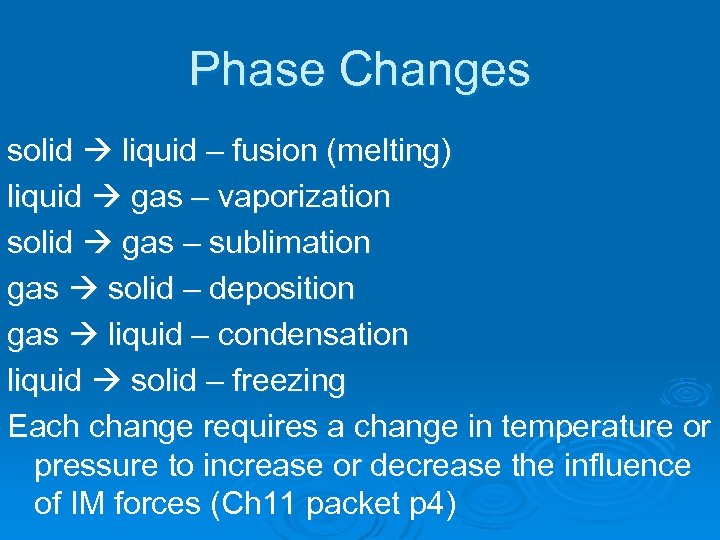Phase Changes solid liquid – fusion (melting) liquid gas – vaporization solid gas – sublimation gas solid – deposition gas liquid – condensation liquid solid – freezing Each change requires a change in temperature or pressure to increase or decrease the influence of IM forces (Ch 11 packet p 4)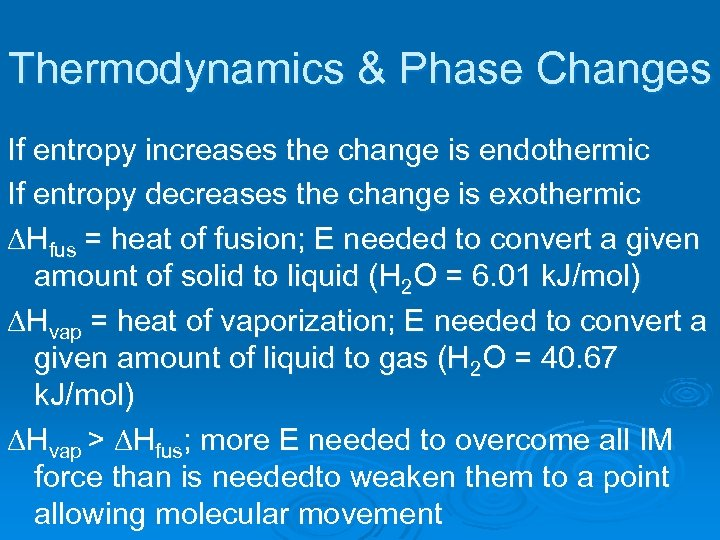Thermodynamics & Phase Changes If entropy increases the change is endothermic If entropy decreases the change is exothermic DHfus = heat of fusion; E needed to convert a given amount of solid to liquid (H 2 O = 6. 01 k. J/mol) DHvap = heat of vaporization; E needed to convert a given amount of liquid to gas (H 2 O = 40. 67 k. J/mol) DHvap > DHfus; more E needed to overcome all IM force than is neededto weaken them to a point allowing molecular movement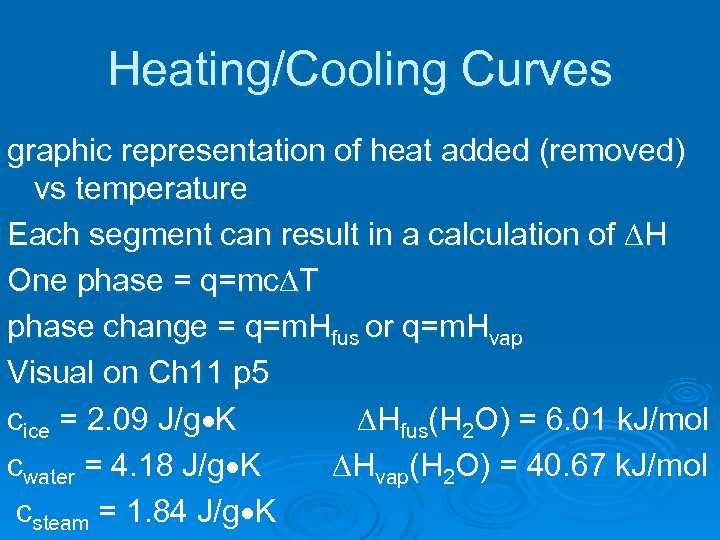Heating/Cooling Curves graphic representation of heat added (removed) vs temperature Each segment can result in a calculation of DH One phase = q=mc. DT phase change = q=m. Hfus or q=m. Hvap Visual on Ch 11 p 5 cice = 2. 09 J/g K DHfus(H 2 O) = 6. 01 k. J/mol cwater = 4. 18 J/g K DHvap(H 2 O) = 40. 67 k. J/mol csteam = 1. 84 J/g K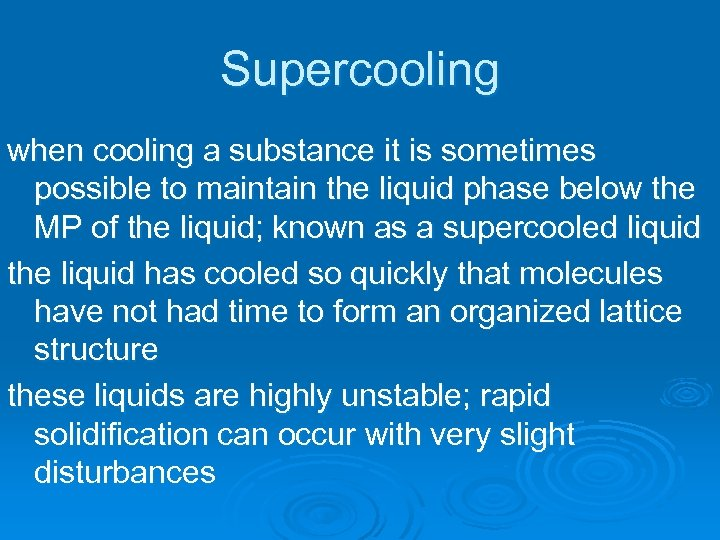Supercooling when cooling a substance it is sometimes possible to maintain the liquid phase below the MP of the liquid; known as a supercooled liquid the liquid has cooled so quickly that molecules have not had time to form an organized lattice structure these liquids are highly unstable; rapid solidification can occur with very slight disturbances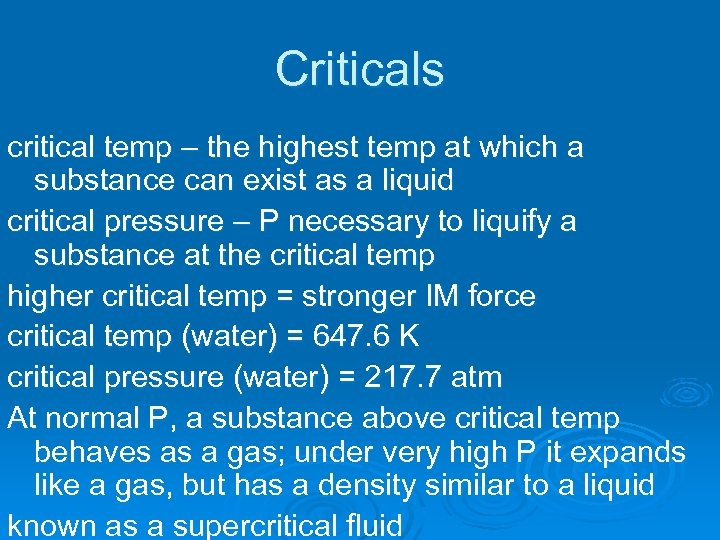Criticals critical temp – the highest temp at which a substance can exist as a liquid critical pressure – P necessary to liquify a substance at the critical temp higher critical temp = stronger IM force critical temp (water) = 647. 6 K critical pressure (water) = 217. 7 atm At normal P, a substance above critical temp behaves as a gas; under very high P it expands like a gas, but has a density similar to a liquid known as a supercritical fluid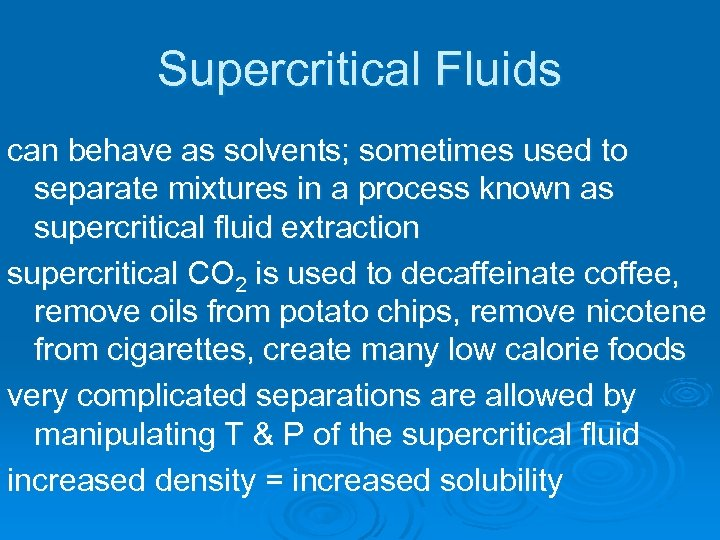Supercritical Fluids can behave as solvents; sometimes used to separate mixtures in a process known as supercritical fluid extraction supercritical CO 2 is used to decaffeinate coffee, remove oils from potato chips, remove nicotene from cigarettes, create many low calorie foods very complicated separations are allowed by manipulating T & P of the supercritical fluid increased density = increased solubility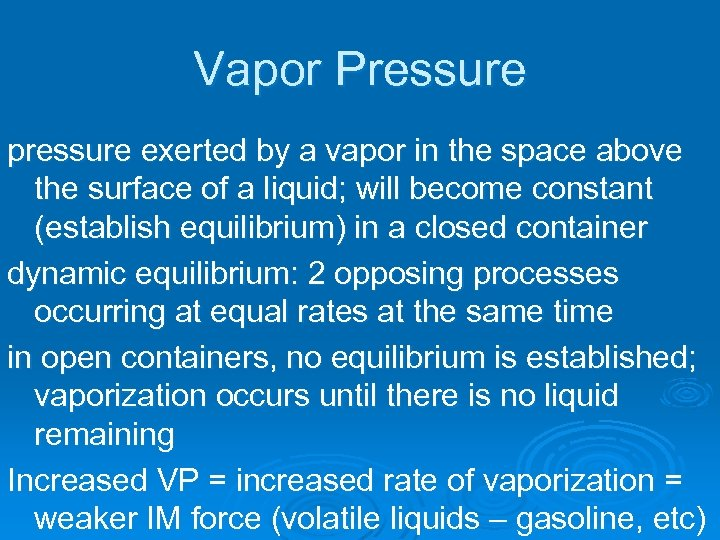Vapor Pressure pressure exerted by a vapor in the space above the surface of a liquid; will become constant (establish equilibrium) in a closed container dynamic equilibrium: 2 opposing processes occurring at equal rates at the same time in open containers, no equilibrium is established; vaporization occurs until there is no liquid remaining Increased VP = increased rate of vaporization = weaker IM force (volatile liquids – gasoline, etc)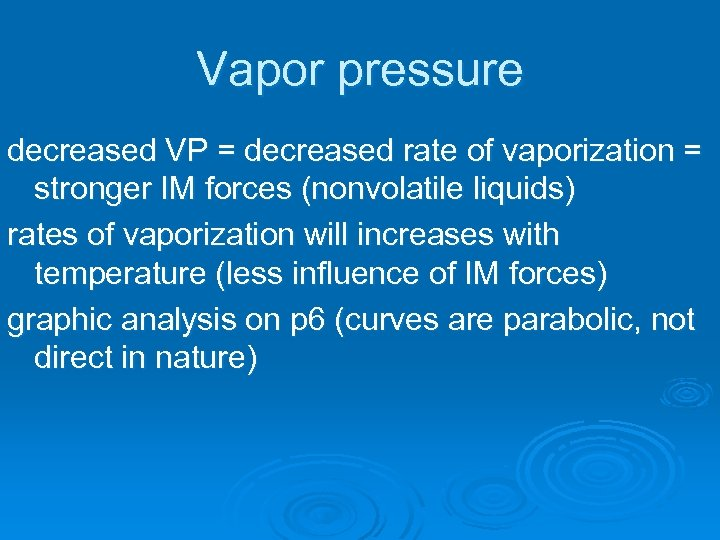Vapor pressure decreased VP = decreased rate of vaporization = stronger IM forces (nonvolatile liquids) rates of vaporization will increases with temperature (less influence of IM forces) graphic analysis on p 6 (curves are parabolic, not direct in nature)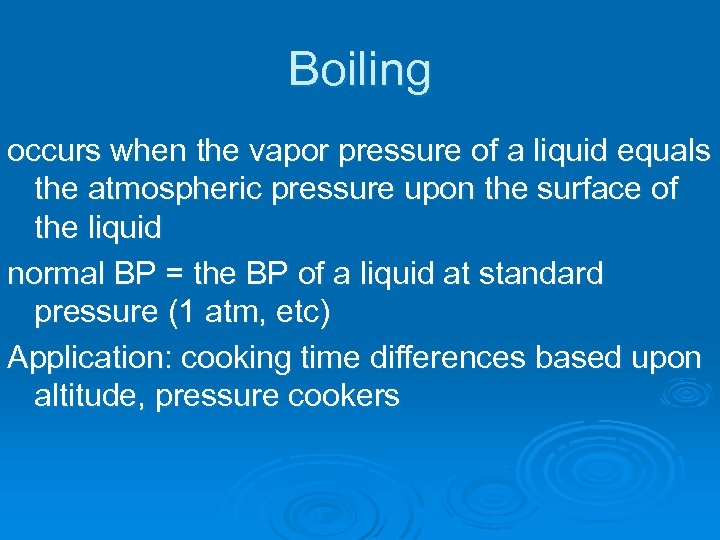Boiling occurs when the vapor pressure of a liquid equals the atmospheric pressure upon the surface of the liquid normal BP = the BP of a liquid at standard pressure (1 atm, etc) Application: cooking time differences based upon altitude, pressure cookers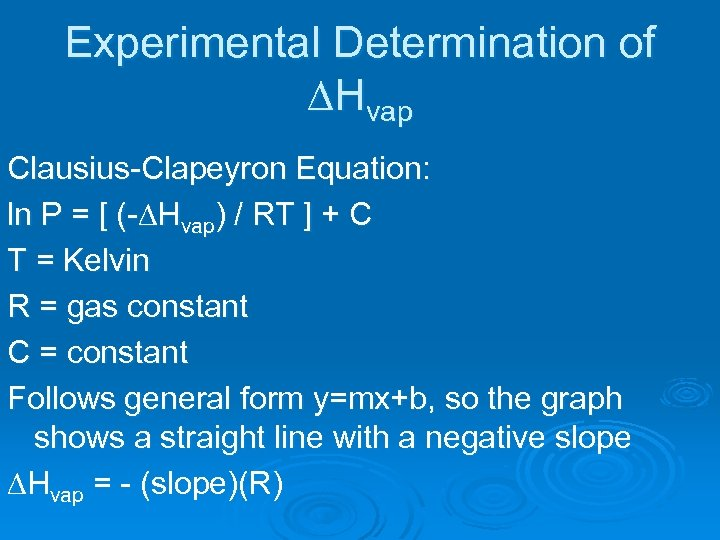Experimental Determination of DHvap Clausius-Clapeyron Equation: ln P = [ (-DHvap) / RT ] + C T = Kelvin R = gas constant C = constant Follows general form y=mx+b, so the graph shows a straight line with a negative slope DHvap = - (slope)(R)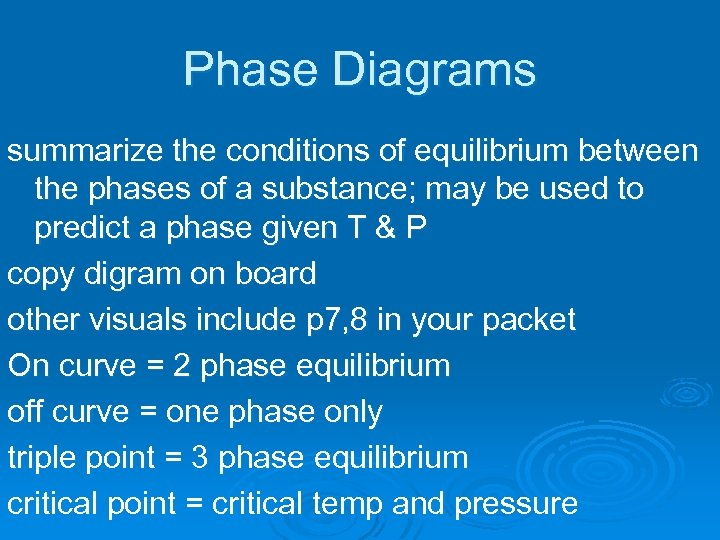Phase Diagrams summarize the conditions of equilibrium between the phases of a substance; may be used to predict a phase given T & P copy digram on board other visuals include p 7, 8 in your packet On curve = 2 phase equilibrium off curve = one phase only triple point = 3 phase equilibrium critical point = critical temp and pressure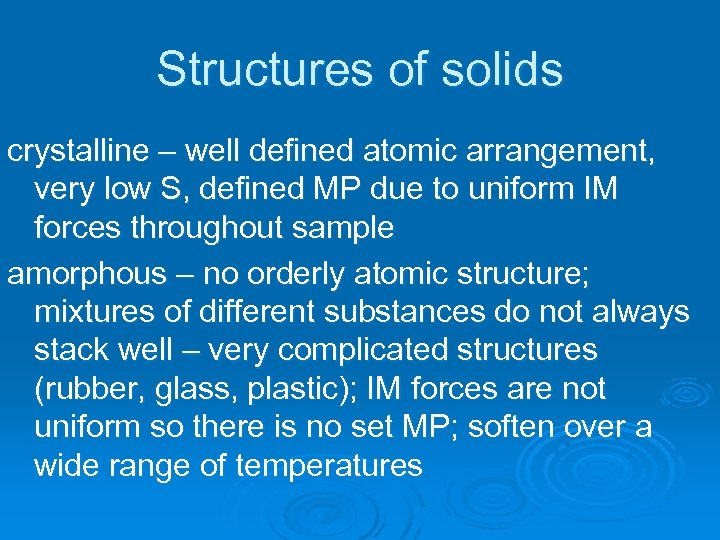Structures of solids crystalline – well defined atomic arrangement, very low S, defined MP due to uniform IM forces throughout sample amorphous – no orderly atomic structure; mixtures of different substances do not always stack well – very complicated structures (rubber, glass, plastic); IM forces are not uniform so there is no set MP; soften over a wide range of temperatures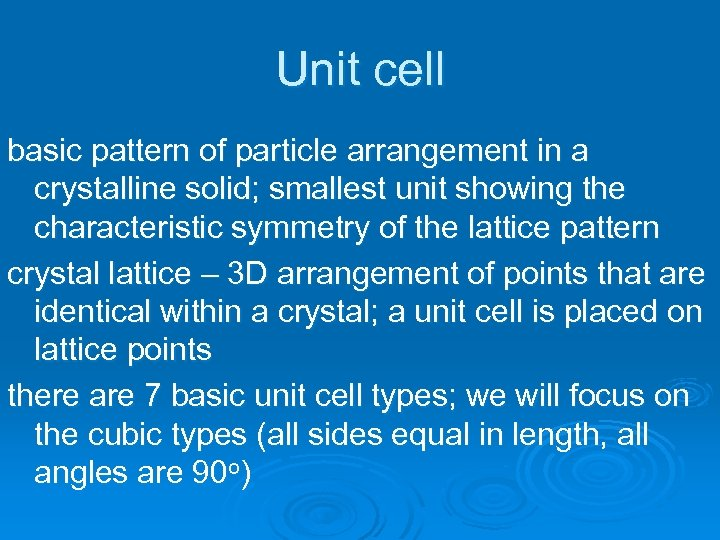Unit cell basic pattern of particle arrangement in a crystalline solid; smallest unit showing the characteristic symmetry of the lattice pattern crystal lattice – 3 D arrangement of points that are identical within a crystal; a unit cell is placed on lattice points there are 7 basic unit cell types; we will focus on the cubic types (all sides equal in length, all angles are 90 o)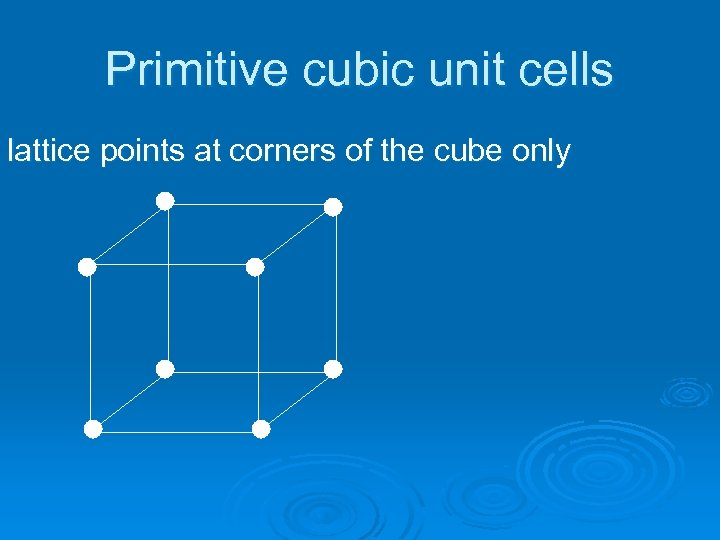Primitive cubic unit cells lattice points at corners of the cube only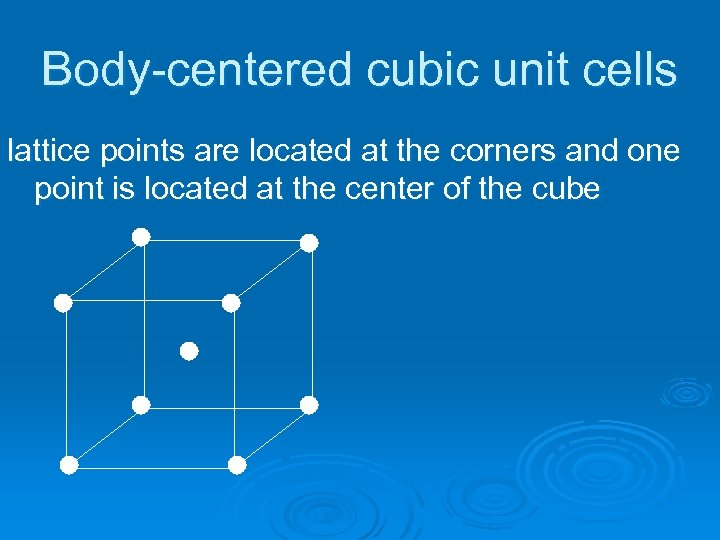Body-centered cubic unit cells lattice points are located at the corners and one point is located at the center of the cube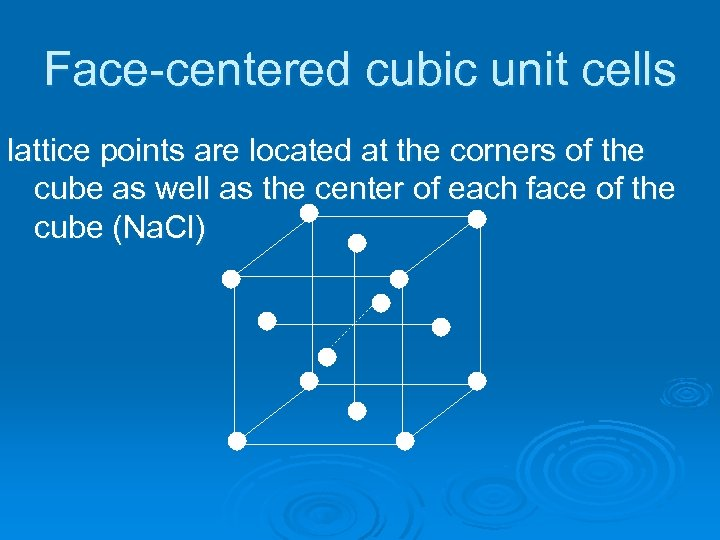Face-centered cubic unit cells lattice points are located at the corners of the cube as well as the center of each face of the cube (Na. Cl)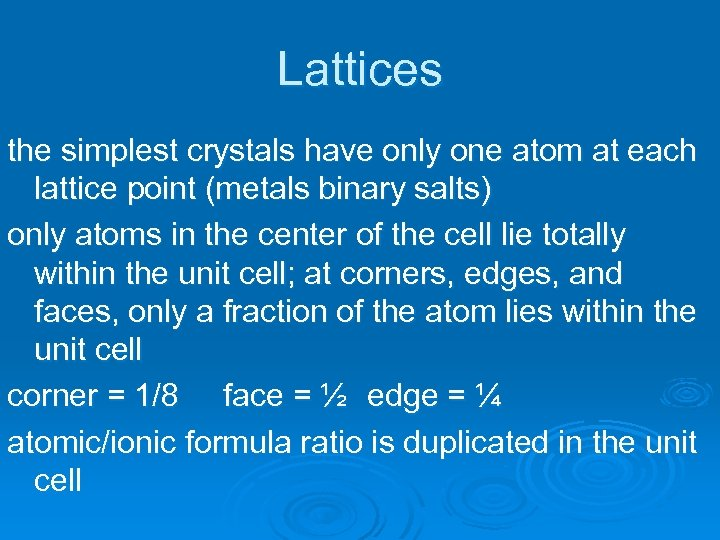Lattices the simplest crystals have only one atom at each lattice point (metals binary salts) only atoms in the center of the cell lie totally within the unit cell; at corners, edges, and faces, only a fraction of the atom lies within the unit cell corner = 1/8 face = ½ edge = ¼ atomic/ionic formula ratio is duplicated in the unit cell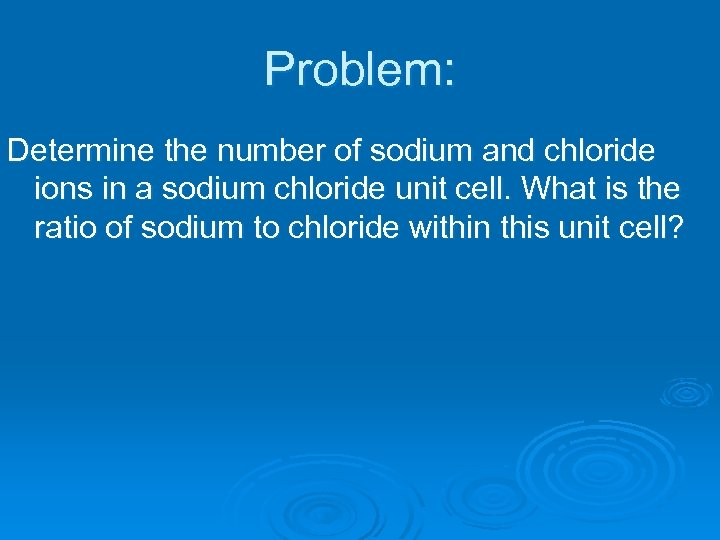Problem: Determine the number of sodium and chloride ions in a sodium chloride unit cell. What is the ratio of sodium to chloride within this unit cell?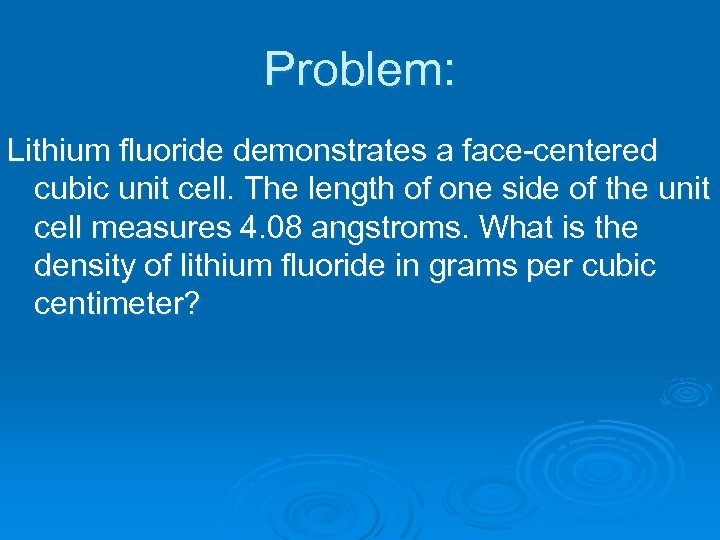Problem: Lithium fluoride demonstrates a face-centered cubic unit cell. The length of one side of the unit cell measures 4. 08 angstroms. What is the density of lithium fluoride in grams per cubic centimeter?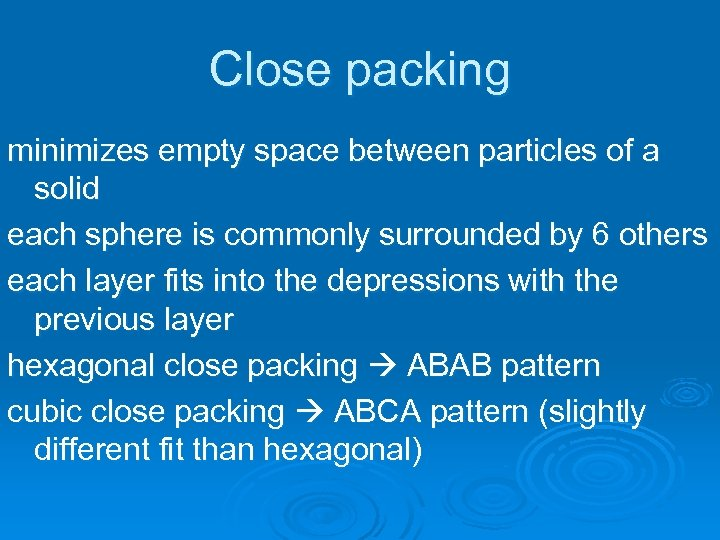Close packing minimizes empty space between particles of a solid each sphere is commonly surrounded by 6 others each layer fits into the depressions with the previous layer hexagonal close packing ABAB pattern cubic close packing ABCA pattern (slightly different fit than hexagonal)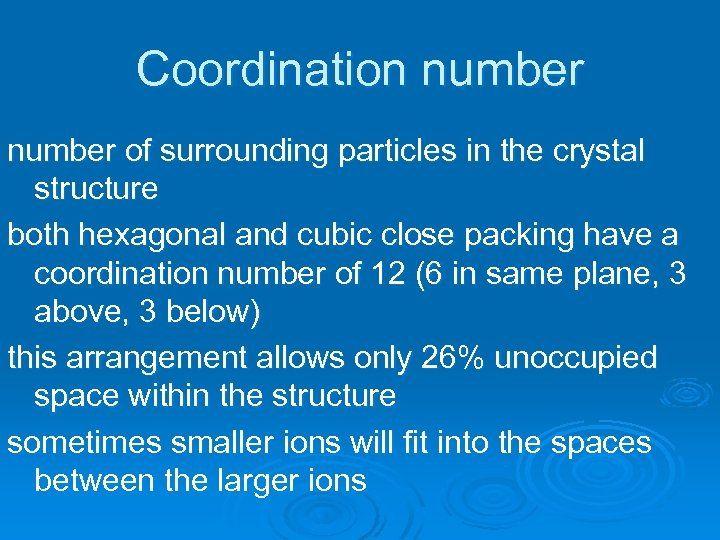Coordination number of surrounding particles in the crystal structure both hexagonal and cubic close packing have a coordination number of 12 (6 in same plane, 3 above, 3 below) this arrangement allows only 26% unoccupied space within the structure sometimes smaller ions will fit into the spaces between the larger ions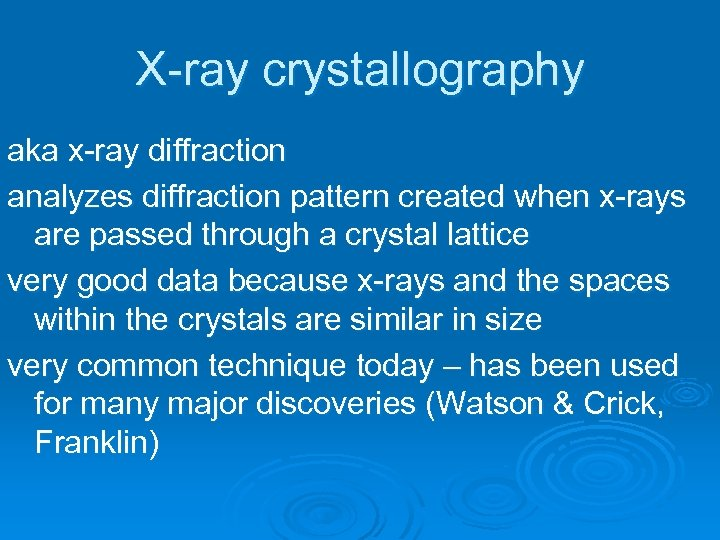X-ray crystallography aka x-ray diffraction analyzes diffraction pattern created when x-rays are passed through a crystal lattice very good data because x-rays and the spaces within the crystals are similar in size very common technique today – has been used for many major discoveries (Watson & Crick, Franklin)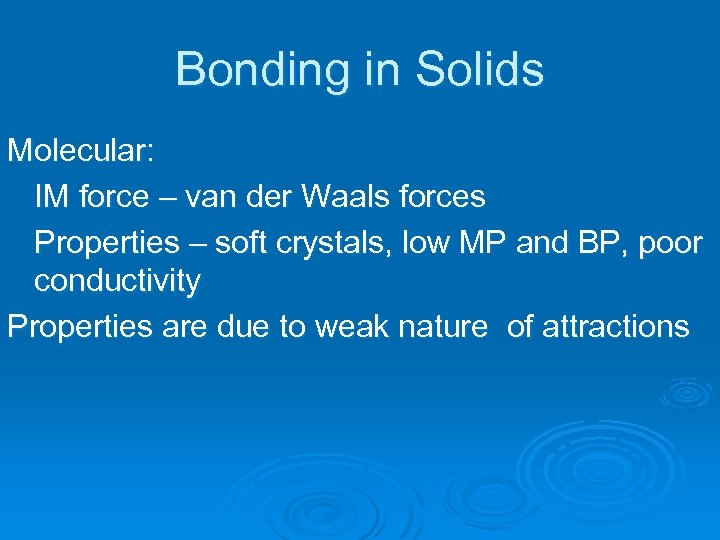Bonding in Solids Molecular: IM force – van der Waals forces Properties – soft crystals, low MP and BP, poor conductivity Properties are due to weak nature of attractions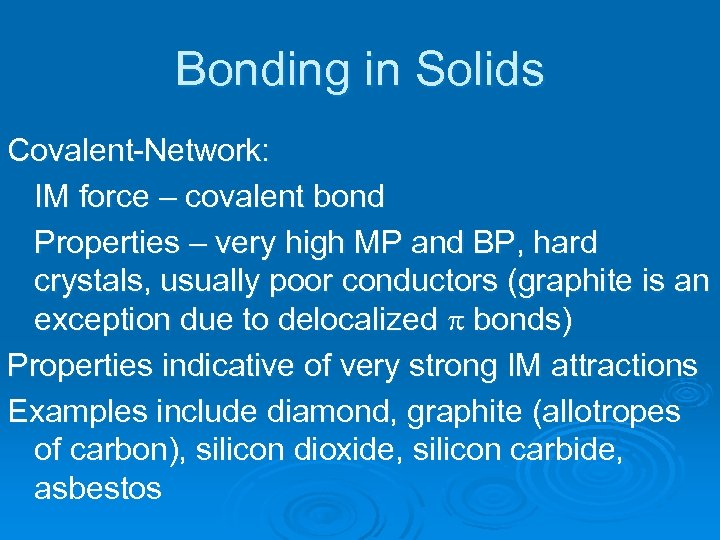Bonding in Solids Covalent-Network: IM force – covalent bond Properties – very high MP and BP, hard crystals, usually poor conductors (graphite is an exception due to delocalized p bonds) Properties indicative of very strong IM attractions Examples include diamond, graphite (allotropes of carbon), silicon dioxide, silicon carbide, asbestos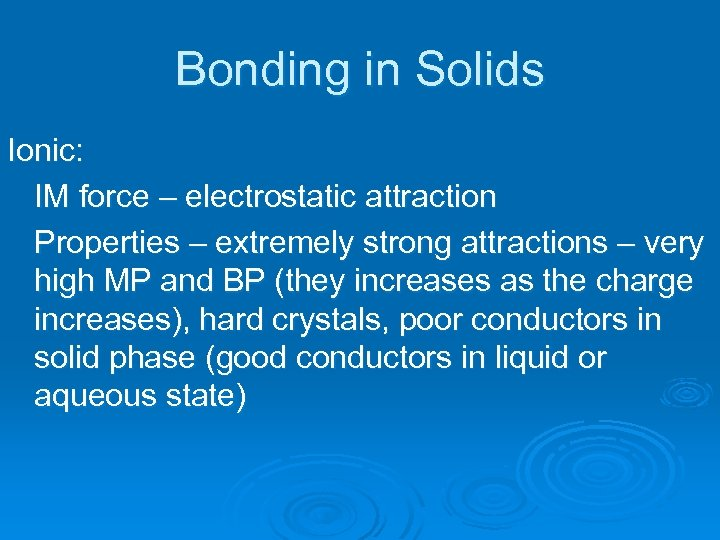Bonding in Solids Ionic: IM force – electrostatic attraction Properties – extremely strong attractions – very high MP and BP (they increases as the charge increases), hard crystals, poor conductors in solid phase (good conductors in liquid or aqueous state)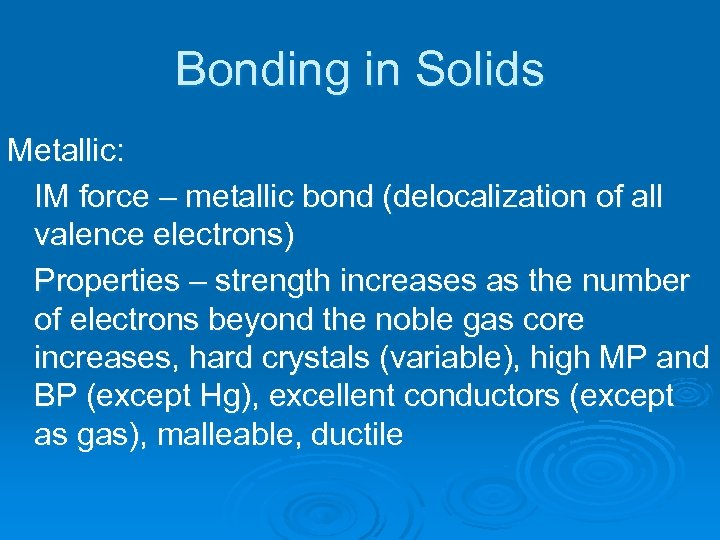Bonding in Solids Metallic: IM force – metallic bond (delocalization of all valence electrons) Properties – strength increases as the number of electrons beyond the noble gas core increases, hard crystals (variable), high MP and BP (except Hg), excellent conductors (except as gas), malleable, ductile